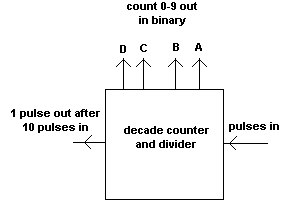Home > Electronic Tutorials > Digital Circuits Tutorial > 7490 Decade Counter Tutorial

Digital Circuits - Electronic TutorialsThe 7490 integrated circuit counts the number of pulses arriving at its input.

The number of pulses counted (up to 9) appears in binary form on four pins of the ic.

When the tenth pulse arrives at the input, the binary output is reset to zero (0000) and a single pulse appears at another output pin.

So for ten pulses in there is one pulse out of this pin.

The 7490 therefore divides the frequency of the input by ten.

If this pulse is applied to the input of a second 7490 then this second ic will count the pulses from the first ic.

It will give one pulse out after 100 pulses have been applied to the first ic.

The 7490 can be connected to divide by other values.

Decimal      Binary
0                0000
1                0001
2                0010
3                0011
4                0100
5                0101
6                0110
7                0111
8                1000
9                1001